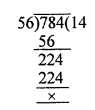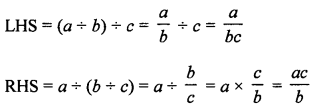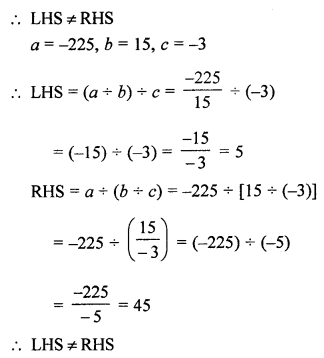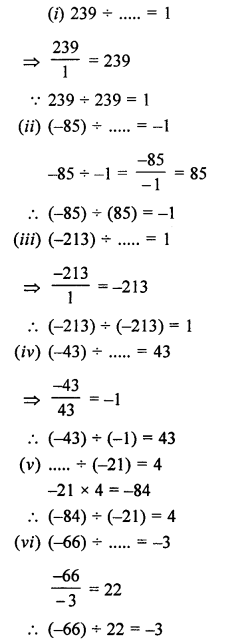## ML Aggarwal Class 7 Solutions for ICSE Maths Chapter 1 Integers Ex 1.4

Question 1.
Evaluate the following:
(i) (-36) ÷ (-9)
(ii) 150 ÷ (-25)
(iii) (-270) ÷ 27
(iv) (-59) ÷ 59
(v) 0 ÷ (-17)
(vi) (-784) ÷ (-56)
Solution:
(i) (-36) ÷ (-9) = $$\frac { -36 }{ -9 }$$ = +4
(ii) 150 ÷ (-25) = $$\frac { 150 }{ -25 }$$ = -6
(iii) (-270) ÷ 27 = $$\frac { -270 }{ 27 }$$ = -10
(iv) (-59) ÷ 59 = $$\frac { -59 }{ 59 }$$ = -1
(v) 0 ÷ (-17) = $$\frac { 0 }{ -17 }$$ = 0
(vi) (-784) ÷ (-56) = $$\frac { -784 }{ -56 }$$ = 14Question 2.
Evaluate the following:
(i) 13 ÷ [(-2) + 1]
(ii) (-47) ÷ [(-45) + (-2)]
(iii) [(-6) + 5] ÷ [(-2) + 1]
(iv) [(-48) ÷ (-6)] ÷ (-2)
Solution:
(i) 13 ÷ [(-2) + 1]
= 13 ÷ [-2 + 1]
= 13 ÷ (-1)
= -13

(ii) (-47) ÷ [(-45) + (-2)]
= (-47) ÷ (-47)
= 1

(iii) [(-6) + 5] ÷ [(-2) + 1]
= [-6 + 5] + [-2 + 1]
= (-1) ÷ (-1)
= 1

(iv) [(-48) ÷ (-6)] ÷ (-2)
= $$\frac { -48 }{ -6 }$$ ÷ (-2)
= 8 ÷ (-2)
= -4

Question 3.
Verify that (a ÷ b) ÷ c ≠ a ÷ (b ÷ c) for a = -225, b = 15 and c = -3.
Solution:Question 4.
Verify that a ÷ (b ÷ c) ≠ (a ÷ b) ÷ (a ÷ c) for
(i) a = -10, b = 1 and c = 1
(ii) a = 12, b = 1 and c = -2
Solution:
a ÷ (b ÷ c) ≠ (a ÷ b) ÷ (a ÷ c)= (12 ÷ 1) + [12 ÷ (-2))
= 12 – 6
= 6
LHS ≠ RHS

Question 5.
Fill in the blanks to make the following statements true:
(i) 239 ÷ …….. = 1
(ii) (-85) ÷ …….. = -1
(iii) (-213) ÷ ….. = 1
(iv) (-43) ÷ …… = 43
(v) …….. ÷ (-21) = 4
(vi) (-66) ÷ ……. = -3
Solution:Question 6.
Write five pairs of integers (a, b) such that a ÷ b = -3 one such pair is (-6, 2).
Solution:
Here, a ÷ b = -3
⇒ a = -3b
Putting some different values to b, we get corresponding values of a such as
(i) b = 1, then
a = -3b = -3 × 1 = -3
(-3, 1)
(ii) b = 3, then
a = -3 × 3 = -9
(-9, 3) is a pair.
(iii) b = 4, then a = -3 × 4 = -12
Pair is (-12, 4)
(iv) b = 5, then a = -3 × 5 = -15
Pair is (-15, 5)
(v) b = 6, then a = -3 × 6 = -18
Pair is (-18, 6)

Question 7.
In a competition 3 marks are given for every correct answer and (-2) marks are given for every incorrect answer and no marks for not attempting any question.
(i) Sachin scored 24 marks. If he got 14 correct answers, how many questions has he attempted incorrectly?
(ii) Nalini scores (-7) marks in this competition, though she has got 9 correct answers. How many questions she has attempted
incorrectly?
Solution:
In a competition,
3 marks for every correct answer and (-2) marks for every incorrect answer.
No marks for non-attempting the question.
(i) Sachin got 14 correct answers, and got 24 marks
He would have got 14 × 3 = 42 marks
But to get 24 marks 42 – 24 = 18 marks were deducted due to incorrect answer.
He attempted $$\frac { 18 }{ 2 }$$ = 9 questions incorrect.
(ii) Nalini scored (-7) marks, she got 9 correct answers, so she would have 9 × 3 = 27 marks
But she got (-7) marks
So, 27 + 7 = 34 marks were deducted due to incorrect answers.
Number of incorrect answers = $$\frac { 34 }{ 2 }$$ = 17 questions

Question 8.
An elevator descends into a mine shaft at the rate of 6 m/min. If the descend starts from 10 m above the ground level, how long will it take to reach the shaft 350 m below the ground level?
Solution:
Rate of elevator = 6 m/min
and it was started 10 m above the ground level
Depth below ground level = 350 m
Total depth = 350 + 10 = 360
Time taken = $$\frac { 360 }{ 6 }$$ minutes = 60 minutes = 1 hour